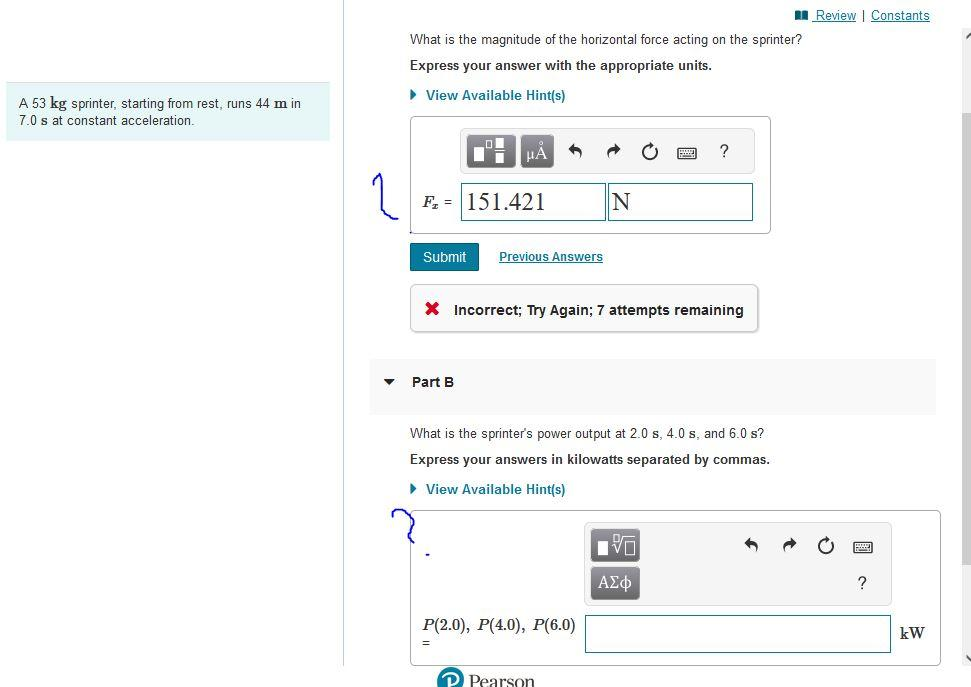# (Solved): What is the magnitude of the horizontal force acting on the sprinter? Express your answer with the ...What is the magnitude of the horizontal force acting on the sprinter? Express your answer with the appropriate units. A sprinter, starting from rest, runs in at constant acceleration. * Incorrect; Try Again; 7 attempts remaining Part What is the sprinter's power output at , and ? Express your answers in kilowatts separated by commas.

We have an Answer from Expert

From Newton's second law, we know the formula of force acting on a moving body is given as

where, m is the mass and a is the acceleration of the body.

In the problem, the mass of the sprinter given,
we can determine the acceleration of the sprinter using the kinematics relation

considering the sprinter has started running from zero initial velocity, i.e.,
and given, the sprinter runs 44m in 7s. So we have x=44m and t=7s
Therefore we can determine the acceleration of the sprinter from the above formula as

The sprinter is having acceleration
We have an Answer from Expert